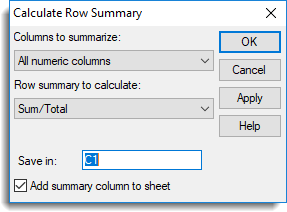1. Home
2. Calculate Row Summary

# Calculate Row Summary

Use this to calculate row summaries within a spreadsheet. Row summaries can be calculated for all variate or factor columns within a spreadsheet.

Spread | Calculate | Row Summaries.
2. Fill in the fields as required then click OK.## Columns to summarize

Lists the type of columns that can be used in forming the row summaries.

 All numeric columns All variate and factor columns within the spreadsheet All variate columns All variate columns within the spreadsheet All factor columns All factor columns within the spreadsheet Selected columns The currently selected columns within the spreadsheet. This option is only available when a spreadsheet contains selected columns. Columns in pointer The columns belonging to a pointer defined within the spreadsheet. This option is only available when a spreadsheet contains pointers. Use the Spread | Sheet | Create pointers menu to define pointers in the spreadsheet.

## Row summary to calculate

The following lists the statistics that can be calculated for each row along with the equivalent function that could be used to perform the same calculation using the CALCULATE directive in the command language.

 Statistic Description Genstat Command Sum/Total The sum/total of the values in the row VTOTALS/VSUMS function Mean/Average The mean of the values in the row VMEANS function Standard deviation The standard deviation of the values in the row VSD function Variance The variance of the values in the row VVARIANCES function Standard error of Means The standard error of the row mean VSEMEANS function Median The median value in the row VMEDIANS function Maxima The maximum value in the row VMAXIMA function Minima The minimum value in the row VMINIMA function Range The range of the values in the row VRANGE function No. of Observations The number of non missing values VNOBSERVATIONS function Percentile The percentile of the values in the row (the percentage should be supplied in the space provided) VPERCENTILES function Quantile The quantile of values in the row (the probability should be supplied in the space provided) VQUANTILES function Position The position of a value in the row (the value to find in each row should be supplied in the space provided. This can be the name of another column in the spreadsheet or a number.) VPOSITIONS function No. of Missing Values The number of missing values (*) VNMV function No. of Values The number of columns VNVALUES function Skewness The skewness of the values VSKEWNESS function Kurtosis The kurtosis of the values VKURTOSIS function

## Percentage (%) (for summary type Percentile only)

A percentage between 0 and 100% for the calculation of the percentile. Percentages of 0, 25, 50 75, and 100 correspond to the minimum, lower quartile, median, upper quartile and maximum data value respectively.

## Probability (for summary type Quantile only)

A probability between 0 and 1 for the calculation of the quantile. Probabilities of 0, 0.25, 0.5, 0.75 and 1 correspond to the minimum, lower quartile, median, upper quartile and maximum data value respectively.

## Find value (for summary type Position only)

The value to find in each row. This can be the name of a variate or a number.

## Save in

The column name to contain the row summaries.